# Colleges with the highest SAT scores in Indiana

Top 10 colleges in Indiana with the highest SAT scores
Looking for the colleges with the highest SAT scores in Indiana? Well you're in luck! We've compiled a national college database and have created a list of the top 10 universities with the highest SAT scores in Indiana. These are the schools whose applicants had the highest average SAT scores in Indiana. And, since these tests are meant to determine academic prowess, they are arguably the schools with the most academically proficient students. You could even say these are the best colleges in Indiana. We also include each college's ACT scores and acceptance rate so that you can see where you would have the easiest or hardest time getting in. Read on to find out more.

## University of Notre Dame SAT scores

The average SAT score for University of Notre Dame is 1475.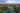The average SAT score of 1475 breaks down into:

• SAT math: 750

The average ACT score for University of Notre Dame is 34 and their acceptance rate is 17.7%.

## Rose-Hulman Institute of Technology SAT scores

The average SAT score for Rose-Hulman Institute of Technology is 1357.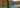The average SAT score of 1357 breaks down into:

• SAT math: 710

The average ACT score for Rose-Hulman Institute of Technology is 30 and their acceptance rate is 60.9%.

## Holy Cross College SAT scores

The average SAT score for Holy Cross College is 1345.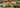The average SAT score of 1345 breaks down into:

• SAT math: 680

The average ACT score for Holy Cross College is 30 and their acceptance rate is 34.2%.

## Purdue University SAT scores

The average SAT score for Purdue University is 1315.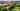The average SAT score of 1315 breaks down into:

• SAT math: 675

The average ACT score for Purdue University is 29 and their acceptance rate is 59.8%.

## Earlham College SAT scores

The average SAT score for Earlham College is 1270.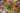The average SAT score of 1270 breaks down into:

• SAT math: 640

The average ACT score for Earlham College is 28 and their acceptance rate is 51.9%.

## DePauw University SAT scores

The average SAT score for DePauw University is 1260.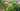The average SAT score of 1260 breaks down into:

• SAT math: 630

The average ACT score for DePauw University is 27 and their acceptance rate is 67.3%.

## Indiana University Bloomington SAT scores

The average SAT score for Indiana University Bloomington is 1255.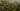The average SAT score of 1255 breaks down into:

• SAT math: 630

The average ACT score for Indiana University Bloomington is 28 and their acceptance rate is 77.9%.

## Butler University SAT scores

The average SAT score for Butler University is 1252.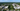The average SAT score of 1252 breaks down into:

• SAT math: 630

The average ACT score for Butler University is 27 and their acceptance rate is 67.8%.

## Wabash College SAT scores

The average SAT score for Wabash College is 1220.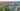The average SAT score of 1220 breaks down into:

• SAT math: 620

The average ACT score for Wabash College is 26 and their acceptance rate is 65%.

## University of Evansville SAT scores

The average SAT score for University of Evansville is 1200.The average SAT score of 1200 breaks down into:

• SAT math: 600